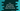# C++ program to print values at odd and even index of an array# Introduction :

In this C++ tutorial, we will learn how to print values at odd and even indices of a user given array. This program will use one integer array. It will read all inputs from the user one by one and it will print all odd and even index values one by one.

I will write the program and explain each step one by one.

## C++ program :

``````#include <iostream>
using namespace std;

int main(int argc, char const *argv[])
{
// 1
int size;
cout << "Enter total number of elements to add : " << endl;
cin >> size;

// 2
int numArray[size];

// 3
for (int i = 0; i < size; i++)
{
cout << "Enter number " << i << " :" << endl;
cin >> numArray[i];
}

// 4
cout << "Odd indexed numbers : " << endl;
for (int i = 0; i < size; i++)
{
// 5
if (i % 2 == 0)
{
continue;
}
cout << "Index " << i << ",value : " << numArray[i] << endl;
}

// 6
cout << "Even indexed numbers : " << endl;
for (int i = 0; i < size; i++)
{
if (i % 2 != 0)
{
continue;
}
cout << "Index " << i << ",value : " << numArray[i] << endl;
}

return 0;
}``````

### Explanation :

The commented numbers in the above program denote the step numbers below :

1. size is an integer variable to hold the size of the array. Ask the user to enter the total number of elements to add and store this value in the size variable.
2. Once we know the count of elements the user is going to enter, create one new array to hold these values. Late initialization of an array helps us to utilize the space.
3. Run one for loop to read the array elements. This loop will ask the user to enter the numbers one by one. It will read each value and store it in the array.
4. Print out all odd indexed values. We are using one for loop to iterate through the array elements. It runs from i = 0 to i = size - 1.
5. Inside the loop, check if the current index or i is even or odd. If it is even, continue i.e. stop the current execution and start the next execution. If it is not even or if it is odd, print the value with the index. The for loop will keep running and all odd indexed values will be printed.
6. Similar to step 4 and 5, print out all even indexed numbers.

### Sample Output :

``````Enter total number of elements to add :
5
Enter number 0 :
2
Enter number 1 :
4
Enter number 2 :
6
Enter number 3 :
8
Enter number 4 :
10
Odd indexed numbers :
Index 1,value : 4
Index 3,value : 8
Even indexed numbers :
Index 0,value : 2
Index 2,value : 6
Index 4,value : 10

Enter total number of elements to add :
4
Enter number 0 :
1
Enter number 1 :
2
Enter number 2 :
3
Enter number 3 :
4
Odd indexed numbers :
Index 1,value : 2
Index 3,value : 4
Even indexed numbers :
Index 0,value : 1
Index 2,value : 3``````

### Conclusion :

If you are not sure about the size of an array, get the size from the user and initialize it. Don’t initialize it with any random high value. It will work but not a good practice for production applications. In this example, we have used one integer array but you can change it to any other type of array. Try to run it and drop one comment below if you have any queries.